Balbharti Maharashtra State Board Class 10 Science Solutions Part 1 Chapter 2 Periodic Classification of Elements Notes, Textbook Exercise Important Questions and Answers.

## Maharashtra State Board Class 10 Science Solutions Part 1 Chapter 2 Periodic Classification of Elements

Periodic Classification Of Elements Question 1.
Rearrange the columns 2 and 3 so as to match with the column 1.

 Column 1 Column 2 Column 3 i. Triad a. Lightest and negatively charged particle in all the atoms 1. Mendeleev ii. Octave b. Concentrated mass and positive charge 2. Thomson iii. Atomic number c. Average of the first and the third atomic mass 3. Newlands iv. Period d. Properties of the eighth element similar to the first 4. Rutherford v. Nucleus e. Positive charge on the nucleus 5. Dobereiner vi. Electron f. Sequential change in molecular formulae 6. Moseley

 Column 1 Column 2 Column 3 i. Triad Average of the first and the third atomic mass Dobereiner ii. Octave Properties of the eighth element similar to the first Newlands iii. Atomic number Positive charge on the nucleus Moseley iv. Period Sequential change in molecular formulae Mendeleev v. Nucleus Concentrated mass and positive charge Rutherford vi. Electron Lightest and negatively charged particle in all the atoms Thomson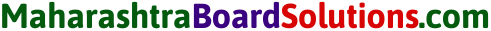Periodic Classification Of Elements Class 10 Maharashtra Board Question 2.
Choose the correct option and rewrite the statement:
(a) The number of electrons in the outermost shell of alkali metals is…….
(a) 1
(b) 2
(c) 3
(d) 7
(a) 1

(b) Alkaline earth metals have valency 2. This means that their position in the modern periodic table is in…….
(a) Group 2
(b) Group 16
(c) Period 2
(d) d-block
(a) Group 2

(c) Molecular formula of the chloride of an element X is XCl. This compound is a solid having high melting point. which of the following elements be present in the same group as X.
(a) Na
(b) Mg
(c) Al
(d) Si
(a) Na

(d) In which block of the modem periodic table are the nonmetals found?
(a) s-block
(b) p-block
(c) d-block
(d) f-block
(b) p-block

Class 10 Science Chapter 2 Periodic Classification Of Elements Notes Question 3.
An element has its electron configuration as 2, 8, 2. Now answer the following questions.
a. What is the atomic number of this element?
The atomic number of this element is 12.

b. What is the group of this element?
The group of this element is 2.

c. To which period does this element belong?
This element belongs to period 3.

d. With which of the following elements would this element resemble? (Atomic numbers are given in the brackets)
N(7), Be(4), Ar(18), Cl(17)
This element resembles Be(4).

Class 10 Science 1 Chapter 2 Periodic Classification Of Elements Question 4.
Write down the electronic configuration of the following elements from the given atomic numbers. Answer the following question with explanation.

a. 3Li, 14Si, 2He, 11Na, 15P which of these elements belong to be period 3?

 Elements Electronic configuration (i) 3Li 2,1 (ii) 14Si 2, 8,4 (iii) 2He 2 (iv) 11Na 2, 8, 1 (v) 15P 2, 8, 5

Elements belong to the 3rd period: 14Si, 11Na and 15P.

b. 1H, 7N, 20Ca, 16S, 4Be, 18Ar. Which of these elements belong to the second group?

 Elements Electronic configuration (i)  1H 1 (ii) 7N 2, 5 (iii) 20Ca 2, 8, 8, 2 (iv) 16S 2, 8, 6 (v) 4Be 2, 2 (iv) 18Ar 2, 8, 8

Elements belongs to the 2nd group: 4Be and 20Ca.

c. 7N, 6C, 8O, 5B, 13Al Which is the most electronegative element among these?

 Elements Electronic configuration (i) 7N 2,5 (ii) 6C 2,4 (iii) 80 2,6 (iv) 5B 2,3 (v) 13A1 2, 8,3

Among these, 8O is the most electronegative element.

d. 4Be, 6C, 8O, 5B, 13Al Which is the most electropositive element among these?

 Elements Electronic configuration (i) 4Be 2, 2 (ii) 6C 2, 4 (iii) 8O 2, 6 (iv) B 2, 3 (v) 11Al 2, 8, 3

Among these, 13Al is the most electropositive element.

e. 11Na, 15P, 17Cl, 14Si, 12Mg which of these has largest atoms?

 Elements Electronic configuration (i) 11Na 2, 8, 1 (ii) 15P 2, 8, 3 (iii) 17Cl 2, 8, 7 (iv) 14Si 2, 8, 4 (v) 12Mg 2, 8, 2

11Na has the largest atomic size.

f. 19K, 3li, 11Na, 4Be Which of these atoms has smallest atomic radius?

 Elements Electronic configuration (i) 19K 2, 8, 8, 1 (ii) 3Li 2, 1 (iii) 11Na 2, 8, 1 (iv) 4Be 2, 2

g. 13Al, 14Si, 11Na, 12Mg, 16S Which of the above elements has the highest metallic character?

 Elements Electronic configuration (i) 13Al 2, 8, 3 (ii) 14Si 2, 8, 4 (iii) 11Na 2, 8, 1 (iv) 12Mg 2, 8, 2 (v) 16S 2, 8, 6

11Na has the highest metallic character.

h. 6C, 3Li, 9F, 7N, 8O Which of the above elements has the highest nonmetallic character?

 Elements Electronic configuration (i) 6C 2, 4 (ii) 3Li 2, 1 (iii) 9F 2, 7 (iv) 7N 2, 5 (v) 8O 2, 6

9F has the highest non metallic character.Science 1 Chapter 2 Class 10 Maharashtra Board  Question 5.
write the name and symbol of the element from the description.
a. The atom having the smallest size.
Helium(He).

b. The atom having the smallest atomic mass.
Hydrogen(H2).

c. The most electronegative atom.
Fluorine(F2).

d. The noble gas with the smallest atomic radius.
Helium(He).

e. The most reactive nonmetal.
Fluorine(F2).

Class 10 Science Chapter 2 Periodic Classification Of Elements  Question 6.
Write short notes.
a. Mendeleev’s periodic law.
when the elements are arranged in the order of their increasing atomic masses, Mendeleev found that the elements with similar physical and chemical properties repeat after a definite interval. On the basis of these finding Mendeleev stated the periodic law. The physical and chemical properties of elements are a periodic function of their atomic masses.

b. Structure of the modern periodic table.
(1) In the modern periodic table, the elements are arranged in the order of their increasing atomic number. In the modern periodic table there are seven horizontal rows called periods and eighteen vertical columns (1 to 18) called groups. The arrangement or the periods and groups results into formation of boxes. Atomic numbers are serially indicated in the upper part of these boxes.

(2) Each box represents the place for one element. Apart from these seven rows, there are two rows of elements placed separately at the bottom of the periodic table. They are lanthanides and actinides series. There are 118 boxes in the periodic table including the two series that means there are 118 places for elements in the modern periodic table.

The formation of a few elements was established experimentally very recently and thereby the modern periodic table is now completely filled with 118 elements.

(3) On the basis of the electronic configuration, the elements in the modern periodic table are divided into four blocks, viz. s-block, p-block, d-block and f-block. The s-block constitute groups 1 and 2. The groups 13 to 18 constitute the p-block. Groups 3 to 12 constitute the d-block, while the lanthanide and actinide series at the bottom form the f-block. The d-bloclçelements are called transition elements.

A zig-zag line shown in the p-block of the periodic table. This zig-zag line shows the three traditional types of elements, i.e. metals, nonmetals and metalloids. The metalloid elements lie along the border of zig-zag line. All the metals lie on the left side of the zig-zag line while all the nonmetals lie on the right side.

c. Position of isotopes in the Mendeleev’s and the modern periodic table.
Isotopes were discovered long time after Mendeleev put forth the periodic table. A challenge was posed in placing isotopes in Mendeleev’s periodic table, as isotopes have the same chemical properties but different atomic masses. Isotopes do not find separate places in this table.

Moseley found out that atomic number is a fundamental property of an element rather than its atomic mass. The atomic number of any element is increased by one unit (number) from the atomic number or subsequent element. In the modern periodic table, the elements are arranged in the order of their increasing atomic numbers, that time the problem or discrepancy in the pairs or isotopes of elements observed in Mendeleev’s periodic table was solved. The isotopes or 17Cl35 and 17Cl37 were placed in the same group as both have the same atomic number.Periodic Classification Of Elements Class 10 State Board Question 7.
Write scientific reasons.
a. Atomic radius goes on decreasing while going from left to right in a period.
(1) In a period while going from left to right, atomic radius goes on decreasing and the atomic number increases one by one, that means positive charge on the nucleus increases by one unit at a time.

(2) However, the additional electron is added to the same outermost shell. Due to the increased nuclear charge the electrons are pulled towards the nucleus to a greater extent, as a result the size of atom decreases i.e., atomic radius decreases.

b. Metallic character goes on decreasing while going from left to right in a period.
(1) Metals have a tendency to lose the valence electrons to form cations. This tendency of an element is called the metallic character of the element.

(2) while going from left to right within a period the outermost shell remains the same and electrons are added to the same shell. However, the positive charge on the nucleus goes on Increasing while the atomic radius goes on decreasing and thus the effective nuclear charge goes on increasing. As a result of this the tendency of atom to lose electrons decreases, i.e., electropositivity decreases. Thus, metallic character goes on decreasing within a period from left to right.

c. Atomic radius goes on increasing down a group.
The size of an atom is indicated by its radius. while going down a group a new shell is added. Therefore, the distance between the outermost electron and the nucleus goes on increasing. These electrons experience lesser pull from the nucleus. Thus, atomic radius goes on increasing down a group.

d. Elements belonging to the same group have the same valency.
(1) The valency of an element is determined by the number of valence electron in the outermost shell of an atom of an element.

(2) All the elements in a group have the same number of valence electrons. Therefore, elements in the same group should have the same valency. For example, the elements of group I contain only one valence electron: the valency of elements of group I is one. Similarly for group II, the valency is two.

e. The third period contains only eight elements even through the electron capacity of the third shell is 18.
(1) In the modern periodic table, there are seven horizontal rows called periods. In a periods elements are arranged in an increasing order of their atomic numbers. The third row contains 8 elements and the electron capacity of the third shell is 18.

(2) In the third period, while moving from left to right, atomic number increases, number of electrons increases in the shell. The number of elements present in 3rd period is decided on the basis of electronic configuration and octet rule.

 Atomic number 11 12 13 14 15 16 17 18 Elements Na Mg Al Si P S Cl Ar

Argon (Ar) is the last element of the third period and has a capacity of maximum 18 electrons. Its octet of electrons is completed and as argon belongs to zero group, the third shell contains 18 electrons.

Periodic Classification Of Elements Exercise Question 8.
Write the names from the description.
a. The period with electrons in the shells, K, L and M.
Third period.

b. The group with valency zero.
Group 18.

c. The family of nonmetals having valency one.
Halogen family.

d. The family of metals having valency one.
Group 1.

e. The family of metals having valency two.
Group 2.

f. The metalloids in the second and third periods.
Boron, Silicon.

g. Nonmetals in the third period.
Phosphorous, sulfur and chlorine and argon.

h. Two elements having valency 4.
Carbon, silicon.Project:
1. Find out the applications of all the inert gases, prepare a chart and display it in the class.
2. Find out the properties and uses of group 1 and group 2 elements.
3. Find out the properties and uses of period 2 and period 3 elements.

Can you recall? (Text Book Page No. 16)

Science 1 Chapter 2 Periodic Classification Of Elements Question 1.
What are the types of matter?
The types of matter are solid, liquid, gas and plasma.

Class 10 Science Chapter 2 Periodic Classification Of Elements Exercise Question 2.
what are the types of elements?
The types of elements are metals, nonmetals and metalloids.

Periodic Classification Of Elements Class 10 Solutions Question 3.
What are the smallest particles of matter called?
The smallest particles are called atoms.

Periodic Classification Of Elements Question 4.
what is the difference between the molecules of elements and compounds?

1. Elements contain only one kind of atoms in the free state or combined state.
2. An element cannot be decomposed into simple substances by any chemical reaction or simple physical process, e.g. copper, iron, oxygen.
3. A compound is produced by a chemical reaction of two or more elements.
4. The constituents of a compound can be separated by a chemical process, e.g. salt, water and sugar.

Can you tell? (Text Book Page No.16)

Identify Dobereiner’s triads from the following groups of elements having similar chemical properties:
(i) Mg (24.3), Ca (40.1), Sr (87.6)
(ii) S (32.1), Se (79.0), Te (127.6)
(iii) Be (9.0), Mg (24.3), Ca (40.1)
(i) S (32.1), Se (79.0), Te (127.6)
(ii) Be (9.0), Mg (24.3), Ca (40.1)

Use your brain power! (Text Book Page No. 20)

Class 10 Periodic Classification Of Elements Solutions Question 1.
write the molecular formulae of oxides of the following elements by referring to the Mendeleev’s periodic table. Na, Si, C, Rb, P, Ba, Cl, Sn.

 Elements Oxides of Elements Na Na2O Sodium oxide Si SiO2 Silicon oxide C CO2 Carbon dioxide Rb Rb2O Rubidium oxide(yellow solid) P P2O5 Phosphorous pentaoxide Ba BaO Barium oxide Cl Cl2O Chlorine monoxide Sn SnO2 Tin oxide(stannic oxide)Periodic Classification Of Elements Class 10 Question 2.
Write the molecular formulae of the compounds of the following elements with hydrogen by referring to the Mendeleev’s periodic table. C, S, Br, AS, F, O, N, Cl.

 Elements Compounds (with hydrogen) C CH4 Methane S H2S Hydrogen sulphide Br HBr Hydrogen bromide As AsH3 Arsine F HF Hydrogen fluoride O H2O Water N NH3 Ammonia Cl HCl Hydrogen chloride

Can you tell? (Text Book Page No. 22)

Periodic Classification Of Elements Class 10 Question And Answers Question 1.
Go through the modern periodic table and write the names one below the other of the elements of group 1.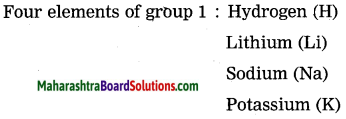Periodic Classification Of Elements Class 10 Short Notes Question 2.
Write the electronic configuration of first four elements in this group.

 Elements Electronic configuration Hydrogen 1 Lithium 2, 1 Sodium 2, 8, 1 Potassium 2, 8, 8, 1

Periodic Classification Of Elements Solutions Question 3.
Which similarity do you find in their configuration?
The similarity is observed in valence electrons of these elements. The valence electron in these elements is one.

10th Science Part 1 Chapter 2 Periodic Classification Of Elements Question 4.
How many valence electrons are there in each of these elements?
There is one valence electron in all these elements.

Can you tell? (Text Book Page No. 23)

Question 1.
On going through the modern periodic table it is seen that the elements Li, Be, B, C, N, O, F and Ne belong to the period-2. write down electronic configuration of all or them.

 Elements Electronic configuration Li 2, 1 Be 2, 2 B 2, 3 C 2, 4 N 2, 5 O 2, 6 F 2, 7 Ne 2, 8

Question 2.
Is the number of valence electrons same for all these elements?
The number of valence electrons is different for all these elements.Question 3.
Is the number of shells the same in these?
The number of shells is the same.

Can you tell? (Text Book Page No. 24)

Question 1.
The elements in the third period, namely, Na, Mg, Al, Si, P, S, Cl and Ar have electrons in the three shells, K, L, M. Write down the electronic configuration of these elements.

 Elements K Shell L Shell M Shell Electronic configuration Na 2 8 1 2, 8, 1 Mg 2 8 2 2, 8, 2 Al 2 8 3 2, 8, 3 Si 2 8 4 2, 8, 4 P 2 8 5 2, 8, 5 S 2 8 6 2, 8, 6 Cl 2 8 7 2, 8, 7 Ar 2 8 8 2, 8, 8

(Think about it) (Text Book Page No.19)

Question 1.
There are some vacant places in Mendeleev’s periodic table. In some of these places, the atomic masses are seen to be predicted. Enlist three of these predicted atomic masses along with their group and period.

 Atomic mass Group Period 44 III 4 72 IV 5 100 VII 6

Question 2.
Due to uncertainty in the names of some of the elements, a question mark is indicated before the symbol in the Mendeleev’s period table. What are such symbols?
Symbols : Yt, Di, Ce, Er, La.

Use your brain power! (Text Book Page No. 19)

Question 1.
Chlorine has two isotopes, viz. cl-35 and Cl-37. Their atomic masses are 35 and 37 respectively. Their chemical properties are same. where should these be placed in Mendeleev’s periodic table? In different places or in the same place?
The arrangement of elements is done on the basis of atomic mass. Since the atomic masses of chlorine (isotopes) are different i.e. 35 and 37, they should be kept in different places in Mendeleev’s periodic table.

Use your brain power! (Text Book Page No.21)

Question 1.
How is the problem regarding the position of cobalt (59CO) and nickel (59Ni) in Mendeleev’s periodic table resolved in modern periodic table?
Mendeleev arranged the elements in their increasing order of atomic masses. But some elements with higher atomic masses are placed before those having lower atomic masses, e.g. cobalt (CO) with atomic mass 58.93 is placed before nickel (Ni) having atomic mass 58.71. Moderm periodic table was prepared on the basis of the atomic number of elements. The atomic number of CO is 27 and that of Ni is 28. So nickel is placed after cobalt.

Question 2.
How thd the position of $${ }_{17}^{35} \mathrm{Cl}$$ get fixed in the modern periodic table?
In Mendeleev’s periodic table, the difference between atomic masses of two consecutive elements is not the same 35Cl and 35Cl. Moseley found out the atomic number of the elements. The atomic number of any element is increased by one unit (number) from the atomic number of subsequent element.

Isotopes $${ }_{17}^{35} \mathrm{Cl}$$ and $${ }_{17}^{37} \mathrm{Cl}$$ occupy the same position in the modern periodic table. Both isotopes have the same atomic number.

In the modern periodic table, the elements are arranged in the order of their increasing atomic numbers, that the problem of discrepancy in the pairs of isotopes elements observed in Mendeleev’s periodic table was solved. The isotopes of $${ }_{17}^{35} \mathrm{Cl}$$ and $${ }_{17}^{37} \mathrm{Cl}$$ were placed in the same group as both have the same atomic number.

Question 3.
Can there be an element with atomie mass 53 or 54 in between the two elements, chromium $${ }_{24}^{52} \mathrm{Cr}$$ and manganese $${ }_{25}^{55} \mathrm{Mn}$$?
In Mendeleev’s periodic table, the difference between atomic masses of two consecutive elements is not the same (52Cr and 55Mn). Moseley found out the atomic number of the elements. The atomic number of any element is increased by one unit (number) from the atomic number of subsequent element. $${ }_{24}^{52} \mathrm{Cr} \rightarrow{ }_{25}^{55} \mathrm{Mn}$$ that means in between two elements (Cr aind Mn), element with mass 53 or 54 do not exist.

Question 4.
what do you think? Should hydrogen be placed in the group 17 of halogens or group 1 of alkali metals in the modern periodic table?
(1) Hydrogen is placed in group 1 and in group 17 as it resembles alkali metals as well as halogens. Thus, no fixed position was given to hydrogen in Mendeleev’s periodic table.

(2) On the other hand, hydrogen easily donates the electron and forms a stable cation (H+), but it does not easily form a stable anion (H), hydride ion. Hence, it is better placed in group 1 rather than in group 17 in the modern periodic table.

Use your brain power! (Text Book Page No. 24)

Question 1.
The elements in the second period : Li, Be, B, C, N, O, F and Ne have electrons in the two shells K and L. Write down the electronic configuration of these elements.

 Element Electronic configuration K shell L shell Li 2 1 Be 2 2 B 2 3 C 2 4 N 2 5 O 2 6 F 2 7 Ne 2 8 Octet complete

Question 2.
The elements in the third period: Na, Mg, Al, Si, P, S, Cl and Ar have electrons in the third shell K, L and M. write down the electronic configuration of these elements.

 Elements K Shell L Shell M Shell Electronic configuration Na 2 8 1 2, 8, 1 Mg 2 8 2 2, 8, 2 Al 2 8 3 2, 8, 3 Si 2 8 4 2, 8, 4 P 2 8 5 2, 8, 5 S 2 8 6 2, 8, 6 Cl 2 8 7 2, 8, 7 Ar 2 8 8 2, 8, 8

Think about it (Text Book Page No. 24)

Question 1.
what is the relationship between the electronic configuration of an element and its valency?
The valency of an element is determined by the number of electrons in the outermost shell.

Question 2.
The atomic number or beryllium is 4. while that of oxygen is 8. Write down the electronic configuration of the two and deduce the valency from the same.

 Element Atomic number Electronic configuration Valency Beryllium 4 2, 2 2 Oxygen 8 2, 6 2

Question 3.
The table given below is based on modern periodic table. write in it the electronic configuration of the first 20 elements below the symbol and write the valency (as shown in a separate box)
Group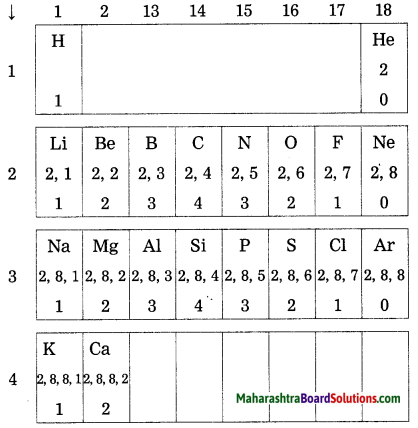Question 4.
what is the periodic trend in the variation of valency while going from left to right within a period? Explain your answer with reference to period 2 and period 3.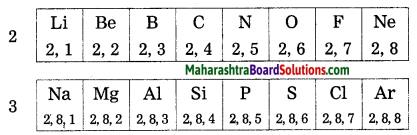(1) In a period, change in valency of an element varies electronic configuration. The number of valence electrons is different in these elements. However, the number of shells is the same.
(2) In a period, while going from left to right, the atomic number increases by one at a time and the number of valence electrons also increases by one at a time.
(3) In periods 2 and 3, while going from left to right, valency varies.

 Elements Li Be B C N O F Ne Valancy 1 2 3 4 3 2 1 0
 Elements Na Mg Al Si P S Cl Ar Valancy 1 2 3 4 3 2 1 0Question 5.
What is the periodic trend in the variation of valency while going down a group?
Explain your answer with reference to the group 1, group 2 and group 18.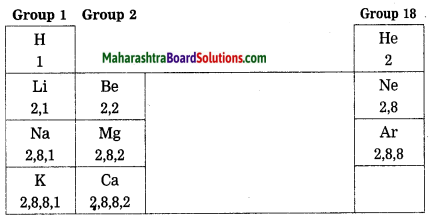(1) The valency of an element is determined by the number of valence electron in the outermost shell of an atom of an element.

(2) All the elements in a group have the same number of valence elements. Therefore, elements in the same group should have the same valency. For example, the elements of group 1 (H, Li, Na, K etc.) contain only one valence electron. the valency of elements of group 1 is one. Similarly for group 2, (Be, Mg, Ca) contain two valence electrons, the valency of elements of group 2 is two.

(3) The elements of group 18 1 (Ne, Ar) contain 8 electrons (exception, Helium contain 2 electrons). Since the octet is completed their valency is zero.

Use your brain power! (Text Book Page No. 25)

 Element K Na Rb Cs Li Atomic radius (pm) 231 186 244 264 152

Question 1.
By referring to the modern periodic table find out the groups to which above the elements belong.
The above elements belong to group 1.

Question 2.
Arrange the above elements vertically downwards in an increasing order of atomic radii.
The above elements arranged vertically downward in an increasing order or atomic radii:

 Li Na K Rb Cs 152 186 231 244 262

Question 3.
Does this arrangement match with the pattern of the group 1 of the modern periodic table?
This arrangement match with the pattern of the group 1 of the modern periodic table in an increasing order of atomic radii.

Question 4.
Which of the above elements have the biggest and the smallest atom?
The biggest atom : Cs
The smallest atom : Li

Question 5.
What is the periodic trend observed in the variation of atomic radii down a group?
while going down a group, atomic number increases, atomic radius increases. Therefore atomic size gradually increases.

Use your brain power! (Text Book Page No. 26)

Question 1.
Look at the elements of third period. Classify them Into metals and nonmetals.
Third row: Na, Mg, Al, Si, P, S, Cl, Ar
Metals: Na, Mg, Al
Nonmetals: P, S, Cl, Ar

Question 2.
On which side of the period are the metals? Left or right?
Left side of the period are the metals.

Question 3.
On which side of the period did you find the nonmetals?
Right side of the period are the nonmetals.

(Use your brain power !) (Text Book Page No. 27)

Question 1.
What is the cause of non-metallic character of elements?
The tendency of an element to form anion or electronegativity is the nonmetallic character of element.

Question 2.
what is the expected trend in the variation of nonmetallic character of elements from left to right in a period?
In a period, as the atomic number increases from left to right, electronegativity increases, non-metallic character increases. This is due to a decrease in the atomic size.

Question 3.
What would be the expected trend in the variation of nonmetallic character of elements down a group?
In a group as the atomic number increases, electropositivity increases while electronegativity decreases, nonmetallic character decreases.

Full in the blanks:

Question 1.
Using Dobereiner’s law of triads, find the missing number.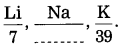Using Dobereiner’s law or triads, the missing number is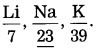Question 2.
In the Mendeleev’s periodic table, properties of elements are periodic function of their ……..
In the Mendeleev’s periodic table, properties of elements are periodic function of their atomic masses.

Question 3.
The vertical columns in the Mendeleev’s periodic table are called …….
The vertical columns in the Mendeleev’s periodic table are called groups.

Question 4.
Eka-aluminium is called ……..
Eka-aluminium is called gallium

Question 5.
Zero group elements are called ……..
Zero group elements are called noble gases.

Question 6.
In the modern periodic table, the elements are the periodic functions of ……..
In the modern periodic table, the elements are the periodic functions of atomic numbers.

Question 7.
The d-block elements are called ……..
The d-block elements are called transition elements.

Question 8.
The group …….. contains the members of the halogen family.
The group 17 halogen the members of the halogen family.

Question 9.
…….. is the distance between the nucleus of the atom and its outermost shell.
Atomic radius is the distance between the nucleus of the atom and its outermost shell.

Question 10.
The number of electrons in an atom is equal to the same of ……..
The number of electrons in an atom is equal to the same of atomic number.

Question 11.
Henry Moseley shows that the atomic number (Z) of an element corresponds to the positive charge on the nucleus or the number of ………
Henry Moseley showed that the atomic number (Z) of an element corresponds to the positive charge on the nucleus or the number of protons.

Question 12.
The ……. block contains the group 1 and 2.
The s-block contains the group 1 and 2.

Question 13.
The elements are arranged in such a way that …… are on left side of zig-zag line and …….. on the right side.
The elements are arranged in such a way that metals are on left side or zig-zag line and nonmetals on the right side.Choose the correct option and rewrite the statement:

Question 1.
(a) Li, Na, K
(b) Ca, Sr, Ba
(c) Be, Mg, Ca
(d) Cu, Ag, Au
(d) Cu, Ag, Au

Question 2.
During Newlands time …….. elements were known.
(a) 56
(b) 65
(c) 63
(d) 36
(a) 56

Question 3.
Halogens belong to group …….. in the modern periodic table.
(a) 15
(b) 16
(c) 17
(d) 18
(c) 17

Question 4.
Noble gases belong to group …….. in modern periodic table.
(a) 15
(b) 16
(c) 17
(d) 18
(d) 18

Question 5.
The law of octaves was given by ……..
(a) Dobereiner
(b) Newlands
(c) Mendeleev
(d) Moseley
(b) Newlands

Question 6.
Eka-boron was subsequently named as ……..
(a) gallium
(b)germanium
(C) scandium
(d) molybdenum
(C) scandium

Question 7.
The halogen which is liquid at room temperature is ………   (Practice 4ctivity Sheet — 3)
(a) fluorine
(b) astatine
(C) bromine
(d) iodine
(C) bromine

Question 8.
……… is used in balloons and in scuba diving.
(a) Helium
(b) Oxygen
(c) Nitrogen
(d) Ozone
(a) Helium

State whether the following statements are True or False. Rewrite the correct statement:

Question 1.
Newlands was the first to classify elements having similar chemical properties into groups
of three.
False. (Dobereiner was the first to classify elements having similar chemical properties into groups of three.)

Question 2.
Dobereiner named the group of elements having similar properties as Triads.
True.

Question 3.
Dobereiner stated the law of octet.
False. (Doberemer stated the law of triads.)

Question 4.
Newlands stated the law of triads.
False. (Newlands stated the law of octaves.)

Question 5.
Eka-aluminium was later named as germanium.
False. (Eka-aluminium was later named as gallium.)

Question 6.
Mendeleev’s periodic table is more useful because it gives information about known and unknown elements.
True.

Question 7.
Mendeleev arranged elements in the increasing order of their atomic masses.
True.

Question 8.
Mendeleev was the first who successfully classified all known elements.
True.

Question 9.
In the modern periodic table, properties of the elements are a periodic function of their atomic numbers.
True.

Question 10.
The d-block elements are called transition elements.
True.

Question 11.
There are 7 periods in the long form of the periodic table.
True.

Question 12.
Elements are classified on the basis of their atomic numbers.
False. (Elements are classified on the basis of their electronic configuration.)

Question 13.
The chemical properties of the elements in the same group show similarity.
True.

Question 14.
Lanthanides and actinides are also called the d-block elements.
False. (Lanthanides and actinides are also called the f-block elements.)

Question 15.
All the elements of a group have the same number of valence electrons.
True.

Question 16.
In a period, atomic sizes increases from left to right.
False. (In a period, atomic size decreases from left to right.)

Question 17.
In a period, the metallic character increases from left to right.
False. (In a period. the metallic character decreases from left to right.)

Question 18.
In a group, the metallic character decreases from top to bottom.
False. (In a group, the metallic character increases from top to bottom.)

Question 19.
The zig-zag line separates the metals from nonmetals in the periodic table i.e. metals are on the left side and nonmetals are on the right side.
True.By observing the correlation in the first pair, complete the second pair:

Question 1.
Dobereiner : Triad : : Newlands law :………
Octaves

Question 2.
Mendeleev’s periodic table : Atomic mass :: Modern periodic table :……..
Atomic number

Question 3.
Group-1 : Alkali metals: :…….: Halogens.
Group 17

Question 4.
Solid : Iodine : : …….. : Bromine.
Liquid

Question 5.
Chlorine : 2, 8, 7 :: Fluorine : ……..
2, 7

Question 6.
Horizontal row Periods : :…….: Groups.
Vertical columns

Find the odd one out and give reasons:

Question 1.
Newlands, Moseley, Dobereiner, Mendeleev.
Moseley. (Moseley brought out the importance of atomic number, while the other tried to classify the elements on the basis or atomic mass.)

Question 2.
Fluorine, Sulphur. Bromine, Iodine.
Sulfur. (Others are halogens.)

Question 3.
Sodium, Aluminium, Chlorine, Carbon.
Carbon. (Carbon belongs to the second row, while the others belong to the third row.)

Question 4.
Nitrogen, Neon, Argon, Helium.
Nitrogen. (The others are inert gases.)Match the columns:

Question 1.

 Column I Column II (1) Modern periodic table (a) Group 17 (2) Vertical columns (b) Period 2 (3) Halogen (c) Atomic number (4) Smallest period (d) Group (e) Period 3

(1) Modern periodic table – Atomic number
(2) Vertical columns Group
(3) Halogen – Group 17
(4) Smallest period – Period 2.

Question 2.

 Column I Column II (1) Dobereiner (a) Atomic number (2) New lands (b) Triads (3) Moseley (c) Atomic mass (4) Mendeleev (d) Octaves (e) Sodium

(2) Newlands – Octaves
(3) Moseley – Atomic number
(4) Mendeleev – Atomic mass.

Question 3.

 Column I Column II (1) Eka-silicon (a) Scandium (2) Eka-boron (b) Gallium (3) Eka-aluminum (c) Germanium (d) Cesium

(1) Eka-silicon – Germanium
(2) Eka-boron – Scandium
(3) Eka-aluminium – Gallium.

Question 4.

 Column I Column II (1) Noble gas (a) 18 elements (2) First period (b) Eight elements (3 ) Second period (c) Two elements (4) 3rd period (d) Helium (e) Six electrons in the last orbit

(1) Noble gas – Helium
(2) First period – Two elements
(3) Second period – Eight elements
(4) 3rd period – 18 elements.

Question 5.

 Column I Column II (1) s-block elements (a) Lanthanides and actinides (2) p-block elements (b) Groups 1, 2 (3) d-block elements (c) Groups IIIA to VIIA and zero group (4) f-block elements (d) Groups 3 to 12 (e) Zero group elements (f) Groups 13 to 18

(1) s-block elements – Groups 1, 2
(2) p-block elements – Groups 13 to 18
(3) d-block elements – Groups 3 to 12
(4) f-block elements – Lanthanides and actinides.

Question 6.

 Column I Column II (1) Helium (a) Alkali metal (2) Horizontal row (b) Alkaline earth metal (3) Group I (c) Period (4) Group II (d) Zero group (e) Metalloid

(1) Helium – Zero group
(2) Horizontal row – Period
(3) Group I – Alkali metal
(4) Group II – Alkaline earth metal.

Write the names from the description:

Question 1.
The period with electrons In the shells K, L and M.
Third period.

Question 2.
The group with valency zero.
Group 18.

Question 3.
The family of nonmetals having valency One.
Halogen family.

Question 4.
The family of metals having valency two.
Group 2.

Question 5.
The metalloids in the second and third period.
Boron, silicon.

Question 6.
The family of metals having valency one.
Group 1.

Question 7.
Nonmetals In the third period.
Phosphorus, sulfur and chlorine, and argon.

Question 8.
Two elements having valency 4.
Carbon. silicon.Question 9.
First three noble gases.
Helium, neon, and argon.

Name the following:

Question 1.
Horizontal rows In modern periodic table.
Periods.

Question 2.
Two elements having a single electron in their outermost shell.
Hydrogen, sodium.

Question 3.
Three elements with filled outermost shell.

1. Helium
2. Neon
3. Argon.

Question 4.
Three elements having 7 electrons in their outermost shell.

1. Fluorine
2. Chlorine
3. Bromine.

Question 5.
An alkali metal in period 2.
Lithium.

Question 6.
An alkaline earth metal in period 3.
Magnesium.

Question 7.
Halogen in period 3.
Chlorine.

Question 8.
Three nonmetallic elements in period 2.

1. Nitrogen
2. Oxygen
3. Fluorine.

Question 9.
The element with electronic configuration (2, 7).
Fluorine.

Question 10.
The elements in periods 2 and 3 having stable electronic configuration.

1. Neon
2. Argon.

Question 11.
The three metals in the third period of the modern periodic table.

1. Sodium
2. Magnesium
3. Aluminum.

Answer the following questions: (Practice Activity Sheet – 3)
An element has its electron configuration as 2,8,8,2.

Question 1.
What is the atomic number of this element?
The atomic number of this element is 20.

Question 2.
what is the group of this element?
The group of this element is 2.

Question 3.
To which period does this element belong?
The element belongs to period 4.

Answer the following questions in one sentence each:

Question 1.
In Dobereiner’s triad containing L, Na, K, if atomic masses of 1ithium and potassium are 6.9 and 39.1, then what will be the atomic mass of sodium? (Practice Activity Sheet – 3) (March 2019)
The atomic mass of sodium is the average of the atomic masses or Li and K i.e., $$\frac{6.9+39.1}{2}$$ = 23.

Question 2.
who was the first scientist to prepare the periodic table?
Mendeleev was the first scientist to prepare the periodic table.Question 3.
State the number of groups and periods in the modern periodic table.
There are 18 groups and 7 periods in the modern periodic table.

Question 4.
How many elements are there in the second and the third periods of the periodic table?
There are eight elements in the second and the third periods of the periodic table.

Question 5.
State the number of elements in the shortest period.
There are two elements in the shortest (first period) period.

Question 6.
State the number of elements in the modern periodic table.
There are 118 elements in the modern periodic table.

Question 7.
which column is known as the zero group in the modern periodic table?
The last column, i.e. 18th column on the right side of the modern periodic table is known as the zero group in the modern periodic table.

Question 8.
which group elements have seven electrons in the outermost shell?
Group 17 elements have seven electrons in the outermost shell.

Question 9.
How many electrons are there in the outermost shell of group 2 elements?
There are 2 electrons in the outermost shell of group 2 elements.

Question 10.
How many electrons are there in the outermost shell of group 18 elements?
There are 8 electrons mn the outermost shell or group 18 elements, except He, which has 2 electrons.

Question 11.
which block or the modern periodic table separates metals and nonmetals with the help of zig-zag line?
p-block of the modern periodic table separates metals and nonmetals with the help of zig-zag line.

Question 12.
Name an alkali metal in the second period.
Lithium is an alkali metal in the second period.

Question 13.
Name the halogen in the second period.
Fluorine is the halogen in the second period.

Question 14.
Name a metalloid in the third period.
Silicon is a metalloid in the third period.

Question 15.
Name the group to which sodium and lithium belong.
Sodium and 1ithium belong to group IA or the periodic table.

Question 16.
Name the group to which magnesium and calcium belong.
Magnesium and calcium belong to group IIA of the periodic table.

Question 17.
Name the group to which the most reactive metals belong.
The most reactive metals belong to group IA.

Question 18.
Name the element having one shell and one valence electron.
Hydrogen has one shell and one valence electron.

Question 19.
How many valence electrons are there in the outermost shell of silicon?
There are four valence electrons present in the outermost shell of silicon.

Question 20.
State the electronic configuration of nitrogen and phosphorus.
Electronic configuration of nitrogen (N) : 2, 5.
Electronic configuration of phosphorus (P) : 2, 8, 5.

Question 21.
write the electronic configuration: 13Al (Practice Activity Sheet – 1)
Electronic configuration of 13Al : 2, 8, 3

Question 22.
Name the group containing highly reactive nonmetals only.
Group 17 contains highly reactive non-metals, namely, fluorine, chlorine, bromine, and iodine.

Question 23.
Name the last three elements of the second period in increasing order of atomic number.
The last three elements of the second period in increasing order of atomic number are oxygen, fluorine, and neon.

Question 24.
Name the three nonmetals in the second period of the modern periodic table.
The three nonmetals in the second period of the modern periodic table are nitrogen, oxygen, and fluorine.

Question 1.
State Doberelner’s law of triads giving one example.
Dobereiner made groups of three elements each, having similar chemical properties and called them triads. He arranged the three elements in a triad in an increasing order of atomic mass and showed that the atomic mass of the middle element was approximately equal to the mean or the atomic masses of the other two elements.
Examples : Lithium (Li), Sodiun (Na), Potassium (K) form Dobereiner’s triad.

Question 2.
Give a suitable illustration of Dobereiner’s law of triads.
(1) Lithium, sodium, and potassium form Doberemer’s triad. They show similar chemical properties. Their atomic masses are as follows:

 Element Li Na K Atomic mass 6.9 23 39.1

According to Dobereiner’s law or triads, the atomic mass of the middle element is approximately the arithmetic mean of the atomic masses of the other two elements.
$$\frac{6.9+39.1}{2}$$ which is approximately the 23.0 atomic mass of sodium.
Thus. the atomic mass of sodium (23) is the average of the atomic masses or lithium (6.9) and potassium 39.1.

(2) Another triad of elements: Calcium (40.1), strontium (87.6) and barium (137.3).

Question 3.
(A, B, C) is a Dobereiner’s triad. complete the following chart and give reason for the answer:

 Element A B C Atomic mass 10.08 12.01 …

 Element A B C Atomic mass 10.08 12.01 13.94

Let the atomic mass of C be x. As (A, B, C) is a Dobereiner’s triad, $$\frac{x+10.08}{2}$$ = 12.01
∴ x = 24.02 – 10.08 = 13.94
∴ atomic mass of C = 13.94.

Question 4.
From the following set of the elements and their atomic masses obtain Dobereiner’s triad:

 Element Br K I Cl Atomic mass 35.5 79.9 126.9 35.5

Among the given four elements, the three elements in the increasing order of atomic masses and having similar properties are

 Element Br K I Atomic mass 35.5 79.9 126.9

Hence, the above three elements represent Dobereiner’s triad.

Question 5.
State the limitations of Dobereiner’s law of triads.

1. During Dobereiner’s period, all elements were not known and also atomic mass was not known accurately.
2. Dobereiner discovered few triads among all the elements.
3. He could not classify aul known elements into triads.Question 6.
State Newlands’ law of octaves.
When the elements are arranged in an increasing order of their atomic masses, the properties of the eighth element are similar to those of the first.
It is found that Na is the eighth element from Li and both of them have similar properties.

Question 7.
Illustrate Newlands’ law of octaves with a suitable example.
(1) Newlands’ law of octaves states that when the elements are arranged in the order of their increasing atomic masses, every eighth element has properties similar to those of the first.

(2) Illustration: If the first 21 elements, except inert gases, are arranged in the order of their increasing atomic masses we have octaves as given below:

 H Li Be B C N O F Na Mg Al Si P S Cl K Ca Cr Ti Mn Fe

It is found that Na is the eighth element from Li and both of them have similar properties. Similarly, the elements, in the following pairs show similar properties: C and Si, Na and K, Mg and Ca, F and Cl.

Question 8.
Explain the limitations of Newlands’ law of octaves.
(1) Newlands’ law of octaves i.e. applicable to only the first few elements i.e., only up to calcium out of total 56 elements known at that time.

(2) Newlands placed two elements each in some boxes to accommodate all known elements e.g. CO and Ni, Ce and La. He placed some elements with different properties under the same note in the octave. For example, Co and Ni under the note Do along with halogens, while Fe having similarity with CO and Ni away from them along with the nonmetals O and S under the note Ti.

(3) Newland’s octaves did not have provision to accommodate the newly discovered elements.

Question 9.
Describe the merits of Mendeleev’s periodic table. (March 2019)
(1) To give the proper place in the periodic table, atomic masses of some elements were revised in accordance with their properties. For example, the previously determined atomic mass or beryllium, 14.09, was changed to the correct value 9.4, and beryllium was placed before boron.

(2) Mendeleev had kept some vacant places in the periodic table for elements that were yet to be discovered. Three of these unknown elements were given the names eka-boron, eka-aluminum and eka-silicon from the known neighbors and their atomic masses were indicated as 44, 68 and 72, respectively. Their properties were also predicted.

Later on, these elements were discovered subsequently and were named as scandium (SC), gallium (Ga) and germanium (Ge) respectively. The properties of these elements matched well with those predicted by Mendeleev. Due to this success all were convinced about the importance of Mendeleev’s periodic table.

(3) There was no place reserved for noble gases in Mendeleev’s original periodic table. when noble gases such as helium, neon and argon were discovered, Mendeleev created the ‘zero group’ without disturbing the original periodic table in which the noble gases were placed very well.

Question 10.
What are the demerits of Mendeleev’s periodic table?
(1) The elements cobalt (CO) and nickel (Ni) have the same whole number atomic mass. As a remit there was an ambiguity regarding their sequence in Mendeleev’s periddic table.

(2) Isotopes were discovered long time after Mendeleev put forth the periodic table. A challenge was posed in placing isotopes in Mendeleev’s periodic table as isotopes have the same chemical properties but different atomic masses.

(3) The rise in atomic mass does not appear to be uniform when elements are arranged in an increasing order of atomic masses. It was not possible, therefore, to predict how many elements could be discovered between two heavy elements.

(4) Position of hydrogen: Hydrogen shows similarity with halogens (group VII). For example, the molecular formula of hydrogen is H2 while the molecular formulae of fluorine and chlorine are F2 and Cl2, respectively. In the same way, there is a similarity in the chemical properties of hydrogen and alkali metals (group I). There is a similarity in the molecular formulae of the compounds of hydrogen alkali metals (Na, K, etc.) formed with chlorine and oxygen. On considering the above properties it is difficult to decide the correct position of hydrogen whether it is in the group of alkali metals (group I) or in the group of halogens (group VII).

 Compounds Of H Compounds Of Na HCl NaCl H2O Na2O H2S Na2S

Similarly in hydrogen and alkali metals.

 Element (Molecular formula) Compounds with metals Compounds with non-metals H2 NaH CH4 Cl2 NaCl CCl4

Question 11.
write a short note on: Moseley’s contribution and the modern periodic table.
The English scientist Henry Moseley demonstrated, with the help of the experiments done using X-ray tube, that the atomic number (Z) of an element corresponds to the positive charge on the nucleus or the number of the protons in the nucleus of the atom or that element. He suggested that ‘atomic number’ is more. fundamental property of an element rather than its atomic mass. On the basis of this research, elements were arranged in the order of their increasing atomic numbers in a more systematic way. Accordingly, the statement of the modern periodic law was stated.Question 12.
State the modern periodic law.
The chemical and physical properties of elements are a periodic function of their atomic numbers.

Question 13.
what is meant by modern periodic table?
The classification of elements resulting from an arrangement of the elements in an increasing order of their atomic numbers (Z) is the modern periodic table.

Question 14.
write the answers to the questions with reference to the structure of the periodic table.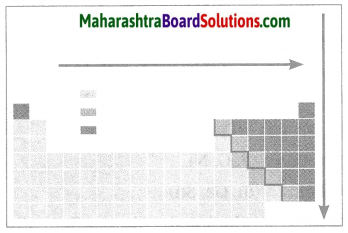(a) Which points are considered for the modern periodic table?
(b) How are blocks indicated?
(c) Which elements are present near the zig-zag line?
(d) Draw the electronic configuration of the second-row elements of first group in the periodic table.
(e) In a periodic table while going from left to right atomic radius decreases. Explain.  (Practice Activity Sheet – 1)
(a) In the modern periodic table, the elements are arranged in the order of their increasing atomic number. In the modern periodic table there are seven horizontal rows called periods and eighteen vertical columns (1to 18) called groups. The arrangement of the periods and groups results into formation of boxes. Atomic numbers are serially indicated in the upper part of these boxes.

(b) On the basis of the electronic configuration, the elements in the modern periodic table are divided into four blocks, viz. s-block, p-block and f-clock, The s-block constitutes the groups 1 and 2. Groups 13 to 18 constitute the p-block. Groups 3 to 12 constitute the d-block, while the lanthanide and actinide series at the bottom form the f-block. The d-block elements are called transition elements. A zig-zag line is shown in the p-block of the periodic table.

(c) The zig-zag line shows the three traditional types of elements, i.e. metals, nonmetals and metalloids. The metalloid elements lie along the border of the zig-zag line. All the metals lie on the left side of the zig-zag line while all the nonmetals lie on the right side.

(d) The electronic configuration of the second row elements of the first group in the periodic table is shown below: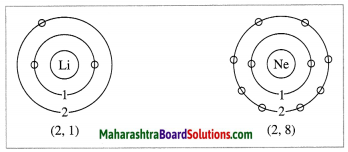(e) (1) In a period while going from left to right, atomic radius goes on decreasing and the atomic number goes on increasing one by one. It means the positive charge on the nucleus increases by one unit at a time.

(2) However, the additional electron gets added to the same outermost shell. Due to the increased nuclear charge the electrons are pulled towards the nucleus to a greater extent. As a result, the size of the atom decreases i.e. the atomic radius decreases.

Question 15.
Observe the figure and answer the following questions.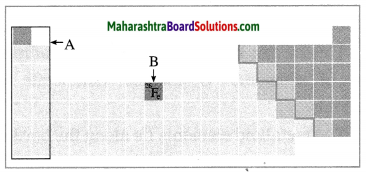(a) Identify the block shown by box A and write an electronic configuration of any one element of this block.
(b) Identify the block of element denoted by letter B and write its period number. (Practice Activity Sheer – 3)
(a) The block shown by box A is the s-block.
Electronic configuration of Mg: 2, 8, 2.

(b) The block of element denoted by letter B is the d-block and its period number is 4.

Question 16.
Give two examples of metalloids.
Metalloids: Boron (B) and Silicon (Si).

Question 17.
write a short note on the zig-zag line in the modern periodic table.

• A zig-zag line is shown in the p-block of the periodic table.
• The zig-zag 1ine shows the three traditional types of elements is metals, nonmetals and metalloids.
• The metalloid elements lie along the border or this zig-zag line.
• All the metals lie on the left side of the zig-zag line.
• All the nonmetal’s lie on the right side of the zig-zag line.

Question 18.
Classify the following elements into group 1, 16 and 17 :
Chlorine, Hydrogen, Oxygen: Bromine.
Group 1 : Hydrogen.
Group 16 : Oxygen.
Group 17 : Chlorine and Bromine.

Question 19.
Classify the following elements into Alkali metals, Halogens, Alkaline earth metals:
(Cl Br I), (Ca, Sr, Mg), (Li, Na, K).
Alkali metals : (Li, Na, K)
Halogens : (Cl Br I).
Alkaline earth metals : (Ca, Sr, Mg).

Question 20.
Classify the following elements into Metals, Nonmetals, Metalloids :
(P, C, N), (Ca, Fe, Al), (Si, Ge, Sn), (K. Mg, Na).
Metals : (Ca, Fe, Al), (K, Mg, Na).
Nonrnetals : (P, C, N).
Metalloids : (Si, Ge, Sn).

Question 21.
Identiry the electronic configuration of the Inert gas elements, third row elements, seventeen group elements, second group elements:
(i) (2, 8, 2), (ii) (2, 8, 8), (iii) (2, 8, 1), (iv) (2, 7), (v) (2, 2), (vi) (2, 8), (vii) (2, 8, 7).
Inert gas elements : (2, 8, 8), (2, 8).
Third row elements : (2, 8, 2), (2, 8, 7), (2, 8, 8).
Second group elements : (2, 8, 2), (2, 2).
Seventeen group elements : (2, 7), (2, 8, 7).

[Note: (1) The outermost shell of all noble gases contain 8 electrons (except He). (2) Atoms or all 3rd row elements contain 3 shells. Out of which first shell contains 2 and 2nd shell contains 8 electrons. (3) The elements of group 17 contains 7 electrons in the outermost shell. (4) The elements or group 2 contains 2 electrons in the outermost shell.]Question 22.
Define : (i) Group (ii) Period.
(i) Group : The vertical column of elements in the periodic table or elements is called a group.
(ii) Period : The horizontal row bf the elements in the periodic able of the elements is called a period.

Question 23.
write the numbers of vertical columns (groups) and horizontal rows (periods) in the long form of the periodic table.
There are 18 vertical columns or groups and seven horizontal rows or periods of the elements in the long form of the periodic table.

Question 24.
Depending on electronic configuration the properties of the elements vary in different groups. Explain why?
(1) There are 18 vertical columns in the modern periodic table and are called groups. These groups are 1 and 2, 13 to 18 and 3 to 12.

(2) The number of valence electrons in all these elements from the group 1, i.e., the family of alkali metals, is the same. Similarly, the elements from any other group, the number of their valence electrons to be the same. For example, the elements beryllium (Be), magnesium (Mg) and calcium (Ca) belong to the group 2, i.e. the family of alkaline earth metals. There are two electrons in their outermost shell the number of valence electrons are 2.

Similarly, there are seven electrons in the outermost shell of the elements such as fluorine (F) and chlorine (Cl) from the group 17, i.e. the family of halogens the number of valence electron is 1. As a result, all elements belonging to the same group have the same valence electrons and show similar chemical properties.

(3) while going from top to bottom within any group, one electronic shell is added at a time. Atomic radius and atomic size increases and hence shows gradation of properties of the elements down the group. From this, the electronic configuration of the outermost shell is characteristic of a particular group.

Question 25.
Depending on electronic configuration the properties of elements vary in different periods. Explain why?
(1) In the modern periodic table, there are seven horizontal rows called periods.

(2) In a period, change in valency of an elements varies electronic configuration.

(3) The number of valence electrons is different in these elements. However, the number of shells is the same. In a period, while going from left to right, the atomic number increases by one at a time and the number of valence electrons also increases by one at a time. In a period, there is gradation in properties of elements.

(4) The elements with the same number of shells occupied by electrons belong to the same period. The elements in the second period, namely, Li, Be, B, C, N, O, F and Ne have electrons in the two shells, K and L. The elements in the third period, namely, Na, Mg, Al, Si, P, S, Cl, and Ar have electrons in the three shell6: K, L and M.

(5) The chemical reactivity of an element is determined by the number of valence electrons in it and the shell number of the valence shell. In a period, while going from left to right, the atomic number increases by one at a time as a result atomic radius gradually decreases. Hence, atomic size decreases.

Question 26.
What is meant by periodic trends in the modern periodic table?
when the properties of elements in a period or a group of the modern periodic table are compared, certain regularity is observed in their variations. it is called the periodic trends in the modern periodic table. The periodic trends are observed in properties of elements, namely, valency, atomic size and metallic-nonmetallic character.

Question 27.
What is meant by valency?
The valency of an element is determined the number of electrons present in the outermost shell of its atoms, i.e. valence electrons.

Question 28.
Define atomic size. How does it vary in a period and a group?
(1) The distance between the centre of the atom and the outermost shell of the atom is called the atomic radius. The size of an atom is indicated by its radius. Atomic radius is expressed in unit picometre (pm). (1 pm = 10-12 m). The size or atom depends on number of shells, more the number of shells larger is the atomic size.

(2) In a group, while going down a group the atomic size goes on increasing because while going down a group newer shells are successively added. This increases the distance between the outermost electron and the nucleus. Hence, the nuclear attraction on these electrons goes on decreasing. Thus in a group atomic size increases.

(3) while going from left to right within a period, atomic radius goes on decreasing and the atomic number goes on increasing one by one. The positive charge on the nucleus increases by one unit at a time. However, the additional electron gets added to the same outermost shell. Due to the increased nuclear charge, the electrons are pulled towards the nucleus to a greater extent, as a result, the size of the atom decreases.

Question 29.
Discuss the trends in the variation of metallic and nonmetallic properties In a period and in a group.
(1) Metals have a tendency to loose the valence electrons to form cations having a stable noble gas configuration. This tendency of an element is called electropositivity is the metallic character of that element.

(2) Nonmetals have a tendency to accept the valence electrons to form anions having a stable noble gas configuration. This tendency of an element is called electronegativity is the nonmetallic character of that element.

(3) In a group, while going down a group a new shell is added, resulting in an increase in the distance between the nucleus and the valence electrons. This results in lowering the effective nuclear charge and thereby lowering the attractive force on the valence electrons. As a result of this the tendency of the atom to lose electrons increases.

Also, the penultimate shell becomes the outermost shell on losing valence electrons. The penultimate shell is a complete octet. Therefore, the resulting cation attains special stability. The metallic character of an atom is its tendency to lose electrons. Therefore, the following trend is observed: The metallic character of elements increases while going down the group.

(4) while going from left to right within a period the outermost shell remains the same. However, the positive charge on the nucleus goes on increasing while the atomic radius goes on decreasing and thus the effective nuclear charge goes on increasing. Therefore, valence electrons are held with greater attractive force. This is called electronegativity. As a result of this the tendency of atom to lose valence electrons decreases within a period from left to right, i.e., electronegativity increases. Thus, non-metallic character of elements increases within a period from left to right.Question 30.
Name the elements, group, formulae and physical state belonging to the halogen family.

 Group Elements Formula Physical state 17 Fluorine F2 Gas Chlorine Cl2 Gas Bromine Br2 Liquid Iodine I2 Solid

Question 31.
Considering the elements of period 3 in the moderm periodic table, answer the following questions:
(a) Name the ‘element’ in which all the shells are completely filled with electrons.Answer:
The element in which all the shells are completely filled with electrons is argon. (2, 8, 8).

(b) Name the element which has one electron in the outermost shell.
The element which has one electron in the outermost shell is sodium (2, 8, 1).

(c) State the most electronegative element in this period.
The most electronegative element in this perod is chlorine (cl).

Question 32.
The atomic number of aluminium is 13. With the help of diagram, write the electronic configuration and valency.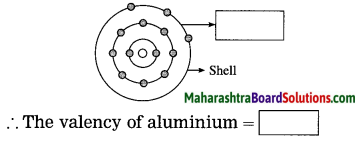The electronic configuration aluminium = 2, 8, 3
The valency of aluminium = 3

Question 33.
Observe the following diagram and answer the following questions: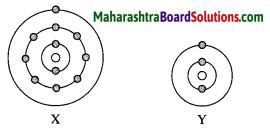(i) Identify elements X and Y.
An element X is Sodium (Na).
An element Y is Lithium (Li).

(ii) Do these elements belong to the same group? Explain.
Yes, these elements belong to the same group as they have the same number of valence electrons.

(iii) which element is more electropositive in nature? Why?
Element X is more electropositive than Y. This is because while going down the group, electropositivity increases with increase in atomic size.

Taking into consideration the period of the elements given below, answer the following questions: (March 2019)

 Element O B C N Be Li Atomic radius (pm) 66 68 77 74 111 152

Question 1.
Arrange the above elements in decreasing order of their atomic radii.
The above elements are arranged in decreasing order of their atomic radii:

 Li Be B C N O 152 111 88 77 74 66

Question 2.
State the period to which the above elements belong.
The above elements belong to period 2.

Question 3.
why this arrangement of elements is similar to the above period of the modern periodic table?
As we move from left to right within a period, the atomic number increases one by one means the position charge on the nucleus increases by one unit at a time, but the electrons are added to the same orbit thereby increasing the pull towards the nucleus which decreases the size of the atom.

Question 4.
Which of the above elements have the biggest and the smallest atom?
The biggest atom: Lithium (Li)
The smallest atom: Oxygen (O)

Question 5.
What is the periodic trend observed in the variation of atomic radius while going from left to right within a period?
while going from left to right in a period, the atomic number increases, atomic radius decreases. Therefore, atomic size gradually decreases.

Write scientific reasons:

Question 1.
Zero group elements (inert gases) are called noble gases.
(1) In the atoms of the inert gas elements (zero group elements), all the electronic shells, including the outermost shell, are completely filled.

(2) The electronic configuration is stable, and these elements do not lose or accept electrons. These elements do not take part in chemical reactions. These elements are gases. Hence, they are called noble gases.

Question 2.
while going down the second group, the reactivity of the alkaline earth metals increases.
The reaction of alkaline earth metal with water is M + 2H2O → M(OH)2 + H2. while going down the second group as Be → Mg → Ca → Sr → Ba, the gradation in this chemical property or the alkaline earth metals is seen. while going down the second group the reactivity of the alkaline earth metals goes on increasing thereby the ease with which this reaction takes place also goes on increasing.

Thus, Beryllium (Be) does not react with water. Mg (Magnesium) reacts with steam. whereas calcium (Ca), strontium (Sr) and barimm (Ba) reacts with water at room temperature with increasing rates.

Question 3.
Fluorine is the most reactive among the halogens.

• Fluorine has the electronic configuration (2, 7).
• It requires only one electron to complete the octet.
• The atomic size of fluorine is the smallest among the halogens. Hence, the nuclear attraction on the outermost electrons is maximum. Hence, fluorine is the most reactive among the halogens.Question 4.
Sodium is more metallic than aluminium.

• Metals give electrons. Sodium has electronic configuration (2, 8, 1). It has only one electron in the outermost shell.
• It can easily give a single electron in the outermost shell. Hence, Sodium is a strong metal.
• Aluminium has an electronic configuration (2,8,3). It has three electrons in the outermost shell.
• Donation of three electrons is more difficult than the donation of one electron. Hence, sodium is more metallic than aluminium.

Distinguish between the following:

Question .1
Mendaleev’s periodic table and Modern periodic table.
Mendaleev’s periodic table:

1. In this table, the elements are arranged in the order of their increasing atomic weights
2. In this table, the position of an element is based on its properties and atomic weight.
3. There are 8 groups in this table.
4. In this table, some elements having similar properties are found in different groups, while those having different properties are sometimes found in the same group.
5. Isotopes do not find separate places in this table.

Modern periodic table:

1. In this table, the elements are arranged in the order of their increasing atomic number.
2. In this table, the position of an element, is based on its electronic configuration.
3. There are 18 groups in this table.
4. In this table, the elements belonging to the same group show similar chemical properties.
5. Isotopes of an element can be placed at the same place as their atomic number is the same.

Question 2.
Groups and periods
Groups:

1. The vertical columns of elements in the modern periodic table are called groups.
2. The group number indicates the number of electrons in the outermost shell of an atom of an element belonging to that group.
3. The elements in the same group show similar chemical properties

periods:

1. The horizontal row of elements in the modern periodic table are called periods.
2. The period number indicates the number of electronic shells present in an atom of an element belonging to that period.
3. The elements in the same period do not show similar properties, but their chemical properties gradually change from left to right in a period.Question 3.
s-block elements and p-block elements